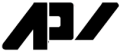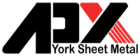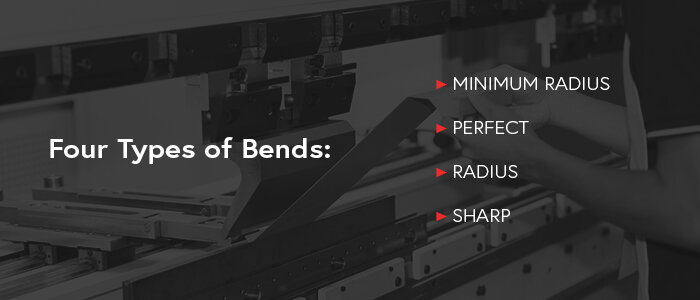## Blog Details## What Is K-Factor in Sheet Metal Bending?

When you’re sheet metal bending, you may need to input a K-factor into your program. You can use standard values or calculate this amount yourself. K-factor plays a key role in understanding the limits a material can handle during sheet metal bending.

## Types of Metal Bends and Forming Methods

Bending and bend formation are important factors when it comes to calculating bend deduction, which is the total elongation for a particular type of bend. The methods used will affect the mathematical values chosen for the calculation.

### 1. METAL BENDING TYPES

When it comes to bending metal, you must consider four types of bends: minimum radius, perfect, radius and sharp. The minimum radius bend moves the metal until its radius is the smallest possible without creating a crease in the material. This value will help you identify perfect, radius and sharp bends.

Perfect bends have values between the minimum radius to 125% of the metal’s thickness. When you surpass 125% of the metal’s thickness, you’ll create a radius bend.

On the other end of the spectrum are sharp bends. These happen when you bend the metal past the minimum radius to form a sharp crease.

### 2. METAL BENDING FORMING

How you bend the metal makes a difference too. You have three main forming methods to choose from — coining, bottom bending and air bending. Among these, coining is the oldest and now mostly obsolete. This method uses a punch nose that presses into the metal and through the neutral radius. In this way, coining thins the material, negatively effecting its integrity.

Bottom bending, while similar to coining, does not ruin the material. The material presses around the punch nose instead of the nose pushing into the metal. The radius of the punch nose determines the radius inside the bend, just as it does with coining.

Air bending does not have a direct correlation between the punch nose radius and the bend radius. Instead, the bend radius is a percentage of the die’s opening. Most engineers favor the 20% rule, which uses percentages around 20% for various metals. For example, for 304 stainless steel, the percentage ranges from 20% to 22%, but for H-series soft aluminum, the range is between 13% and 15%.

## What Are K- and Y-Factors?

K-factor and Y-factor in sheet metal both look at how bending affects the material and how much bending the metal will allow. When sheets of metal bend, the top surface compresses and the bottom expands. The boundary inside the metal between these two is the neutral radius. In flat metal, this boundary evenly bisects the material’s thickness, but it shifts when you bend the metal.

The K-factor comes from the ratio of the neutral radius divided by the thickness of the material on prepared charts and has a value between 0.3 and 0.5. The Y-factor looks at a similar value, but it takes the stresses inherent in the material into consideration, making it more accurate than the K-factor.

## How to Calculate K- and Y-Factors

You can calculate K-factor and Y-factor for various metals, but they will require more involved math when you’re doing them by hand rather than using the simple ratio found in reference materials.

### 1. HOW TO DETERMINE K-FACTOR

If you want to find the K-factor value on your own, you’ll need to take some measurements with several pieces of metal. You must know the inside radius in addition to the dimensions before and after bending. You can use gauge pins and radius gauges to find these values or try an optical comparator. First, use this information to find the bend allowance, BA. (BA = total of inside formed dimensions – flat dimensions.)

Next, measure the inside radius, Ir, and the complementary bend angle, which is 180 degrees minus the included angle. Find the material thickness, Mt, and you’ll have the information you need for calculating the K-factor for sheet metal bending.

To find the K-factor, divide the product of 180 and BA by the difference between the product of pi, Mt and the complementary bend angle to the ratio of Ir to Mt. Mathematically, this formula looks like this:

K-factor = {(180 x BA) ÷ [(π x Complementary Bend Angle x Mt) – (Ir ÷ Mt)]}

### 2. FINDING Y-FACTOR FOR SHEET METAL BENDING

You’ll need the K-factor to find the Y-factor. To calculate Y-factor, you should multiply K-factor by pi and divide the result by two.

Y-factor = (K-factor x π) ÷ 2

## How K- and Y-Factors Affect Metal Bending

K- and Y-factors make metal bending more precise without damaging the material. Once you know the K-factor you can identify the location of the neutral axis after bending in addition to how much the material elongates during bending.

## Factoring in K- and Y-Factors When Designing Custom Metal Parts

Because not all materials have K-factors or Y-factors on a chart, you can calculate these values on your own with your particular choice of metal. Using the aforementioned calculations, you won’t need to rely on preset values when you’re customizing your own designs. This ability will allow you to branch out from standards on tables.

When you’re calculating bend deduction, you can use the K-factor or Y-factor. The bend deduction comes from the bend allowance and the outside setback, OSSB. Multiply the outside setback by two and subtract the bend allowance from that product to find the bend deduction, BD. The OSSB is the tangent of the bend angle divided by two times the sum of the inside radius and the material thickness.

BD = (2 x OSSB) – BA

OSSB = tan (bend angle ÷ 2) x (Ir + Mt)

Recall that BA is the difference between the total formed dimensions and the flat dimensions. Another way to calculate this value is with the K-factor or Y-factor.

With the K-factor, the BA is equal to the following: BA = {[(π ÷ 180) x Ir] + [(π ÷ 180) x K-factor] x Mt}

With the Y-factor, the BA is equal to the following: BA = [(π ÷ 2) x Ir + (Y-factor x Mt)]

With these formulas, you can use your calculated K-factor and Y-factor to find the bend deduction.

## Contact APX York Sheet Metal for Custom Metal Fabrication

If you need custom metal fabrication solutions, turn to the team at APX York Sheet Metal. Though we specialize in metal bending, we can create any customization you need for your parts. Large or small projects don’t matter to us — we take orders of all sizes and will work completely in-house to complete your project.

For a turnkey solution, trust our more than 71 years in the industry for your custom metal fabrication project. Contact us today at APX York Sheet Metal for a free quote.

### Why aluminum is one of the most popular metal?

Aluminum is one of the most popular metals used throughout a variety of industries because, when fabricated the right way, it offers a wide range

### The Importance of Sandblasting Before Powder Coating

Sandblasting is one of the most important steps you can take when powder coating metal parts. It’s an essential part of cleaning metal before powder

### How Maintenance of Laser Cutting Machine Affects Quality

Machine maintenance is a vital part of a laser cutter’s performance. While companies or users may know they need to maintain their machinery, they may

### Smart Manufacturing Technology

Smart technology is everywhere — our homes, cars, smartphones and offices. Shopping experiences are tailored to our exact interests, fast food restaurants have started using

### How Does Laser Cutting Work?

Laser cutting is used in everything from manufacturing to surgery. With its many applications, laser cutting is crucial to the success of many companies. If